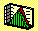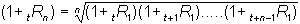2.12 CHAPTER 2: EXERCISES

1. The following table provides the total return for each year from 1980 to 1993 for the Australian All Ordinaries stock index and an index constructed from Property Trusts listed on the Australian Stock Exchange (ASX).

 Year Ended All Ordinaries Return Property Trust Return 1980 48.9% 6.4% 1981 -12.9% 32.1% 1982 -13.9% 5.2% 1983 66.8% 50.2% 1984 -2.3% 10.1% 1985 44.1% 5.2% 1986 52.2% 35.4% 1987 -7.9% 5.7% 1988 17.9% 16.1% 1989 17.4% 2.3% 1990 -17.5% 8.7% 1991 34.2% 20.1% 1992 -2.3% 7.0% 1993 45.4% 30.1%

Source: Property Investor August, 1994.

a) Compute the sample mean return for each index over the period provided.

b) Assume that you invest \$1 at the beginning of 1980 in each index. Construct for each year the end of year value for each \$1 investment, assuming that no distributions or additions are made. That is at the end of 1981 the dollar invested in the all ordinaries index will grow to:

\$1 x 1.489 x 0.871 = \$1.297

Record for each year and for each index the end of year value for your investment.

c) In general define the geometric average (annualized) return, tRn, from an n year investment commencing at the present time t, as follows:That is, if the \$1 investment is compounded each year at the product of the one plus 1 year realized rates of return, then the geometric average return is the average rate of compounding over the investment horizon.

Using your results from part b), compute the average rate of compounding for each \$1 investment over the 14 years.

d) Which investment, in part b), do you think has the greatest risk? Provide qualitative reasons supporting your choice.

e) Using the table of returns compute the unbiased standard deviation of returns for each index.

f) Using your answer to e) as a quantitative measure of risk, is this measure consistent with your qualitative answer to d)? If so, describe a set of circumstances where these two answers will not be consistent. If not, describe what is not captured by the quantitiative measure in e).

g) Compute the sample correlation between the returns from each index.

2. Using the table of returns provided in exercise 1, assume that you invest 50% of your wealth in the All Ordinaries index and 50% of your wealth in the ASX Property Trust Index. Compute the following portfolio statistics:

a) The portfolio mean.

b) The portfolio variance.

3. Assume that for two stocks, ABX and BCA that a financial analyst forecasts the current year's return distribution as follows:

 % Chance ABX BCA Pessimistic 30 -.15 -.05 Most Likely 50 .12 .06 Optimistic 20 .21 .15

Assume that the pessimistic, most likely, and optimistic state is independent across stocks. That is, if the pessimistic return is realized for ABX then any return is still possible for BCA.

a) Compute the expected return from each stock.

b) Compute the variance-covariance matrix of returns for the two stocks.

c) Compute the expected return and portfolio volatility for the following three portfolios:

i. 25% invested in ABX and 75% invested in BCA.

ii. 50% invested in ABX and 50% invested in BCA.

iii. 75% invested in ABX and 25% invested in BCA.

previous topic

next topic

(C) Copyright 1999, OS Financial Trading System